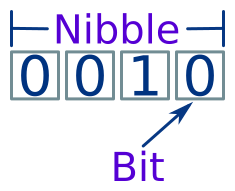# Bits and bytes

Martin McBride, 2016-11-21
Tags bit byte memory nibble word hexadecimal
Categories data representation binary numbers

In everyday life we use denary, or base 10 numbers. Denary uses digits 0 to 9.

Computers use binary(base 2) numbers. Binary only uses digits 0 and 1.

## Bits

A bit represents a single binary digit ("bit" is short for binary digit).

It can have a value 1 or 0, but in a computer program this value can be used to represent true/false, yes/no, on/off.

A bit is the smallest piece of information a computer can store.

## Bytes

A byte is a group of 8 bits:A byte can be used to represent an 8 digit binary number. 011000102 (binary) is equivalent to 98 denary.

A byte can take any value between 0 and 255 (0x00 to 0xFF in hexadecimal. There 256 possible values:A byte can also be used to represent a character (letter, digit or punctuation symbol) using the ASCIIcoding. It can be used to represent an instruction in a machine code computer program.

## Bytes and memory

Computer memory is organised in bytes. A byte is the smallest amount of data that a computer can read from (or write to) memory. You cannot read or write individual bits in memory.

In a similar way, files are also organised in bytes.

## Nibbles

A nibble is similar to a byte, but has 4 bits rather than 8:A nibble has 16 possible values, and can represent integer values from 0 to 15.

In hexadecimal notation, a nibble is a single digit 0x0 to 0xF.

The term nibble isn't used much these days. Some of the first microprocessors developed in the 1970s used 4 bits nibbles rather than 8 bits bytes, due to cost and technical limitations. They are still used in some cases where a very simple, very low cost processor is required.

## Words

Two bytes can be combined to create a 16 bit word. A word has 65536 possible values, and can represent values from 0 to 65,535 (0xFFFF in hex).Four bytes can be combined to create a 32 bit word. This is sometimes called double word or DWORD. It can represent values up to 4,294,967,296 or 0xFFFFFFFF in hex.

Eight bytes can be combined to create a 64 bit word. This is sometimes called long word or LWORD. It can represent values up to about 18 billion billion, or 0xFFFFFFFFFFFFFFFF in hex.

The terms word, double word and long word are not well defined, for example sometimes “word” refers to a 32 bit word.